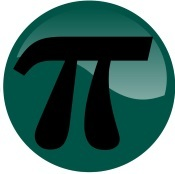• Browse All Lessons

Assign Lesson

Help Teaching subscribers can assign lessons to their students to review online!

 Tweet

Irrational NumbersIntroduction: Up to now, most of the numbers you have used in math have been rational numbers. Rational numbers are the numbers you are familiar with -- whole numbers, integers, or any number that can be written as a ratio of two integers (fraction). When the fraction is divided, its decimal expansion either ends or repeats. However, there are numbers where this does not occur. These are irrational numbers.

• An irrational number cannot be written as a ratio of two integers (fraction).
• An irrational number goes on forever. Its decimal expansion has no pattern and does not end.

Irrational Number Examples
Irrational NumberDecimal Approximation
$pi$$3.1416...$
$sqrt2$$1.4142...$
$sqrt3$$1.7320...$
$e$$2.7182...$
At first, this may seem a bit bewildering when trying to solve a math problem. Fortunately, irrational numbers are written with symbols. In addition, we can approximate the value of irrational numbers. The table shows some examples of irrational numbers.

Required Videos:

Related Worksheets: